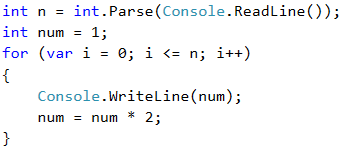# Example: Numbers from 1 to 2^n with a For Loop

In the following example, we will look at using the usual step with size of 1, combined with a calculation at each loop iteration.

Write a program that prints the numbers from 1 to 2^n (two in power of n). For example, if n = 10, the result will be: 1, 2, 4, 8, 16, 32, 64, 128, 256, 512, 1024.

## Video: Numbers 1 ... 2^n

Watch this video lesson to learn how to iterate over the number from 1 to 2^n using a for-loop: https://youtu.be/B2k_yx3EV0I.

## Hints and Guidelines

The code below demonstrates how we can calculate the powers of 2 for given n using a for-loop with a calculation at the end of its body: